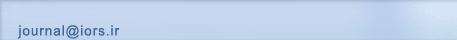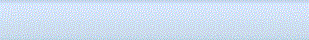[Home ] [Archive]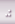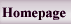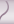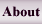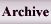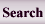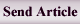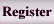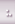Home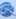Journal InformationArticles archiveSubmission InstructionRegistrationSubmit articleSite FacilitiesContact us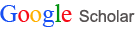All Since 2016 Citations 49076 16489 h-index 9 6 i10-index 9 4

Search in website
 Search the articlesSearch the site Advanced Search
 Enter your Email in the following box to receive the site news and information.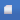Search published articles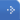Showing 18 results for Algorithm

Emilio Spedicato, Marco Bonomi, Antonino Del Popolo,
Volume 1, Issue 1 (5-2008)
Abstract

Abstract

We consider an application of the ABS procedure to the linear systems arising from the primal-dual interior point methods where Newton method is used to compute path to the solution. When approaching the solution the linear system, which has the form of normal equations of the second kind, becomes more and more ill conditioned. We show how the use of the Huang algorithm in the ABS class can reduce the ill conditioning. Preliminary numerical experiments show that the proposed approach can provide a residual in the computed solution up to sixteen orders lower.

Volume 2, Issue 2 (6-2011)
Abstract

We consider the maximal covering location-allocation problem with multiple servers. The objective is to maximize the population covered, subject to constraints on the number of service centers, total number of servers in all centers, and the average waiting time at each center. Each center operates as an M/M/k queuing system with variable number of servers. The total costs of establishing centers and locating servers should not exceed a predetermined amount. We present a mathematical model for the problem, and propose a heuristic solution procedure with two local search algorithms for improving the solutions. Finally, some computational results are presented.

Sheibani,
Volume 2, Issue 2 (6-2011)
Abstract

We describe a hybrid meta-heuristic algorithm for combinatorial optimization problems with a specific reference to the travelling salesman problem (TSP). The method is a combination of a genetic algorithm (GA) and greedy randomized adaptive search procedure (GRASP). A new adaptive fuzzy a greedy search operator is developed for this hybrid method. Computational experiments using a wide range of standard benchmark problems indicate that the proposed hybrid meta-heuristic approach is very efficient.

Etebari, Aaghaie, Khoshalhan,
Volume 3, Issue 1 (4-2012)
Abstract

In recent years, enriching traditional revenue management models by considering the customer choice behavior has been a main challenge for researchers. The terminology for the airline application is used as representative of the problem. A popular and an efficient model considering these behaviors is choice-based deterministic linear programming (CDLP). This model assumes that each customer belongs to a segment, which is characterized by a consideration set, which is a subset of the products provided by the firm that a customer views as options. Initial models consider a market segmentation, in which each customer belongs to one specific segment. In this case, the segments are defined by disjoint consideration sets of products. Recent models consider the extension of the CDLP to the general case of overlapping segments. The main difficulty, from a computational standpoint, in this approach is solving the CDLP efficiently by column generation. Indeed, it turns out that the column generation subproblem is difficult on its own. It has been shown that for the case of nonoverlapping segments, this can be done in polynomial time. For the more general case of overlapping segments, the column generation sub-problem is NP-hard for which greedy heuristics are proposed for computing approximate solutions. Here, we present a new approach to solve this problem by using a genetic algorithm and compare it with the column generation method. We comparatively investigate the effect of using the new approach for firm’s revenue
Lalwani, Kumar, Spedicato, Gupta,
Volume 3, Issue 1 (4-2012)
Abstract

We present an application of ABS algorithms for multiple sequence alignment (MSA). The Markov decision process (MDP) based model leads to a linear programming problem (LPP), whose solution is linked to a suggested alignment. The important features of our work include the facility of alignment of multiple sequences simultaneously and no limit for the length of the sequences. Our goal here is to avoid the excessive computing time, needed by dynamic programming based algorithms for alignment of a large number of sequences. In an attempt to demonstrate the integration of the ABS approach with complex mathematical frameworks, we apply the ABS implicit LX algorithm to elucidate the LPP, constructed with the assistance of MDP. The MDP applied for MSA is a pragmatic approach and entails a scope for future work. Programming is done in the MATLAB environment
Dr. Behrouz Kheirfam,
Volume 4, Issue 1 (5-2013)
Abstract

We present a new full Nesterov and Todd step infeasible interior-point algorithm for semi-definite optimization. The algorithm decreases the duality gap and the feasibility residuals at the same rate. In the algorithm, we construct strictly feasible iterates for a sequence of perturbations of the given problem and its dual problem. Every main iteration of the algorithm consists of a feasibility step and some centering steps. We show that the algorithm converges and finds an approximate solution in polynomial time. A numerical study is made for the numerical performance. Finally, a comparison of the obtained results with those by other existing algorithms is made.

A.m. Bagirov,
Volume 5, Issue 1 (5-2014)
Abstract

Here, an algorithm is presented for solving the minimum sum-of-squares clustering problems using their difference of convex representations. The proposed algorithm is based on an incremental approach and applies the well known DC algorithm at each iteration. The proposed algorithm is tested and compared with other clustering algorithms using large real world data sets.
Volume 6, Issue 1 (3-2015)
Abstract

‎It is a well-known fact that finding a minimum dominating set and consequently the domination number of a general graph is an NP-complete problem‎. ‎In this paper‎, ‎we first model it as a nonlinear binary optimization problem and then extract two closely related semidefinite relaxations‎. ‎For each of these relaxations‎, ‎different rounding algorithm is exploited to produce a near-optimal dominating set‎. ‎Feasibility of the generated solutions and efficiency of the algorithms are analyzed as well‎.

Msr. Raheleh Taghavi, Dr. Mohammad Ranjbar,
Volume 6, Issue 2 (9-2015)
Abstract

Air defense is a crucial area for all naval combat systems. In this study, we consider a warship equipped with an air-defense weapon that targets incoming threats using surface-to-air missiles. We define the weapon scheduling problem as the optimal scheduling of a set of surface-to-air missiles of a warship to a set of attacking air threats. The optimal scheduling of the weapon results in an increase in the probability of successful targeting of all incoming threats. We develop a heuristic method to obtain a very fast and acceptable solution for the problem. In addition, a branch and bound algorithm is developed to find the optimal solution. In order to increase the efficiency of this algorithm, a lower bound, an upper bound and a set of dominance rules have been developed. Using randomly generated test problems, the performance of the proposed solution approaches is analyzed. The results indicate that in all practical situations, the branch-and-bound algorithm is able to solve the problem optimally in less than a second.

Dr. Behrouz Kheirfam,
Volume 6, Issue 2 (9-2015)
Abstract

In this paper, we propose an arc-search corrector-predictor
interior-point method for solving \$P_*(kappa)\$-linear
complementarity problems. The proposed algorithm searches the
optimizers along an ellipse that is an approximation of the central
path. The algorithm generates a sequence of iterates in the wide
neighborhood of central path introduced by Ai and Zhang. The
algorithm does not depend on the handicap \$kappa\$ of the problem,
so that it can be used for any \$P_*(kappa)\$-linear complementarity
problem. Based on the ellipse approximation of the central path and
the wide neighborhood, we show that the proposed algorithm has
\$O((1+kappa)sqrt{n}L)\$ iteration complexity, the best-known
iteration complexity obtained so far by any interior-point method
for solving \$P_*(kappa)\$-linear complementarity problems.

Prof. Kourosh Eshghi, Mr. Mohsen Salarrezaei,
Volume 7, Issue 1 (4-2016)
Abstract

First, an integer programming model is proposed to find an α-labeling for quadratic graphs. Then, a Tabu search algorithm is developed to solve large scale problems. The proposed approach can generate α-labeling for special classes of quadratic graphs, not previously reported in the literature. Then, the main theorem of the paper is presented. We show how a problem in graph theory can be modeled and solved by an integer programming model and a metaheuristic approach.

Volume 8, Issue 1 (4-2017)
Abstract

We present a bi-objective model for a green truck scheduling and routing problem at a cross-docking system. This model determines three key decisions at the cross dock: (1) defining a sequence and schedule of inbound trucks at the receiving door, (2) specifying a sequence and a schedule of outbound trucks at the shipping door, and (3) determining the routes of the outbound truck while serving customers. The first objective function is related to responsiveness of the network that minimizes time window violations and the second objective function minimizes total fuel consumption of trucks in order to consider the environmental factor of the network. Also, a learning effect is considered in loading and unloading process times. To solve the bi-objective model, an archived multi-objective simulated annealing (AMOSA) is used and modified. Finally, a number of test problems are solved and the efficiency of the proposed AMOSA is compared with the e-constraint method.

Dr. Adil Bagirov, Dr. Sona Taheri,
Volume 8, Issue 2 (5-2017)
Abstract

Clustering problems with the similarity measure defined by the \$𝐿_1\$-norm are studied. Characterizations of different stationary points of these problems are given using their difference of convex representations. An algorithm for finding the Clarke stationary points of the clustering problems is designed and a clustering algorithm is developed based on it. The clustering algorithm finds a center of a data set at the first iteration and gradually adds one cluster center at each consecutive iteration. The proposed algorithm is tested using large real world data sets and compared with other clustering algorithms.
Dr. M. Niksirat,
Volume 9, Issue 1 (7-2018)
Abstract

In this paper bus scheduling problem under the constraints that the total number of buses needed to perform all trips is known in advance and the energy level of buses is limited, is considered. Each depot has a different time processing cost. The goal of this problem is to find a minimum cost feasible schedule for buses. A mathematical formulation of the problem is developed. When there are two depots, a polynomial time algorithm is developed for the problem and theoretical results about the complexity and correctness of the algorithm is presented. Also, several examples are introduced for illustrating validity of the algorithm.
Dr. Reza Ghanbari, Mrs. Effat Sadat Alavi,
Volume 9, Issue 1 (7-2018)
Abstract

A new integer program is presented to model an independent resources assignment problem with resource shortages in the context of municipal fire service. When shortage in resources exists, a critical task for fire departmentchr('39')s administrator in a city is to assign the available resources to the fire stations such that the effect of the shortage to cover (in providing service, in extinguishing fire and so on) is minimized. To solve the problem, we propose a polynomial time greedy algorithm.
Dr Zhang Wei, Prof. Cornelis Roos,
Volume 9, Issue 2 (6-2018)
Abstract

We deal with a recently proposed method of Chubanov , for solving linear homogeneous systems with positive variables. We use Nesterovchr('39')s excessive gap method in the basic procedure. As a result, the iteration bound for the basic procedure is reduced by the factor \$nsqrt{n}\$. The price for this improvement is that the iterations are more costly, namely \$O(n^2 )\$ instead of \$O(n)\$. The overall gain in the complexity hence becomes a factor of \$sqrt{n}\$.
Mrs. Mahnaz Naghshnilchi,
Volume 10, Issue 1 (7-2019)
Abstract

Capacitated vehicle routing problem (CVRP) is one of the most well-known and applicable issues in the field of transportation. It has been proved to be an NP-Complete problem. To this end, it is needed to develop a high-performance algorithm to solve the problem, particularly in large scales. This paper develops a novel mathematical model for the CVRP considering the satisfaction level of demand nodes. Then, the proposed model is validated using a numerical example and sensitivity analyses that are implemented by CPLEX solver/GAMS software. To solve the problem efficiently, a Genetic Algorithm (GA) is designed and implemented. The obtained results demonstrate that the proposed GA can yield high-quality solutions compared to exact solutions.
Mrs. Firozeh Bastan, Dr. Seyyed Mohamad Taghi Kamel Mirmostafaee,
Volume 10, Issue 2 (9-2019)
Abstract

Here, we work on the problem of point estimation of the parameters of the Poisson-exponential distribution through the Bayesian and maximum likelihood methods based on complete samples. The point Bayes estimates under the symmetric squared error loss (SEL) function are approximated using three methods, namely the Tierney Kadane approximation method, the importance sampling method and the Metropolis-Hastings within Gibbs algorithm. The interval estimators are also obtained. The performance of the point and interval estimators are compared with each other by means of a Monte Carlo simulation. Several conclusions are given at the end.

 Page 1 from 1